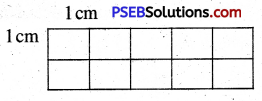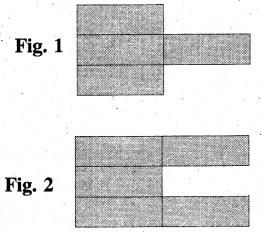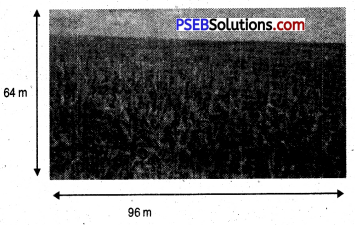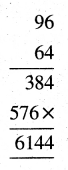# PSEB 5th Class Maths MCQ Chapter 8 Perimeter and Area

Punjab State Board PSEB 5th Class Maths Book Solutions Chapter 8 Perimeter and Area MCQ Questions and Answers.

## PSEB 5th Class Maths Chapter 8 Perimeter and Area MCQ Questions

Multiple Choice Questions

Tick (✓) the right answer :

Question 1.
Which type of figure is notebook’s page ?
(a) Square
(b) Rectangle
(c) Triangle
(d) Pentagon.
(b) RectangleQuestion 2.
What is the perimeter of the square if its side is 6 cm ?
(a) 36 cm
(b) 18 cm
(c) 24 cm
(d) 21 cm.
(c) 24 cm

Question 3.
The four sides of square are
(a) different
(b) equal
(c) two equal pairs
(d) none.
(b) equal

Question 4.
The length and breadth of rectangle is 6 m and 4 m. Find its perimeter.
(a) 36 m
(b) 16 m
(c) 20 m
(d) 10 m.
(c) 20 mQuestion 5.
A rectangular park is 65 m long and 35 m wide. Mukesh takes 4 rounds of it. How much distance is covered by him ?
(a) 100 m
(b) 200 m
(c) 400 m
(d) 800 m.
(d) 800 m

Question 6.
What will be the area of a square whose side is 13 cm ?
(a) 169 cm
(b) 169 sq. cm
(c) 52 sq. cm
(c) 26 sq. cm.
(a) 169 cm

Question 7.
A chart is 125 cm long and 8 cm wide. Its area = ………………..
(a) 100 sq. cm
(b) 1000 sq. cm
(c) 1250 sq. cm
(d) 1100 sq. cm.
(b) 1000 sq. cmQuestion 8.
If length and breadth of the rectangle is equal then it is called ………………
(a) Rectangle
(b) Length
(c) Square
(d) Perimeter.
(c) Square

Question 9.
Side × Side is the area of a ………………
(a) Square
(b) Rectangle
(d) Circle.
(a) Square

Question 10.
Area of a rectangle is 96 sq. cm. If its length is 12 cm then its breadth is :
(a) 8 cm
(b) 9 cm
(c) 10 cm
(d) 108 cm.
Ans.
(a) 8 cmQuestion 11.
Find the area of given rectangle.(a) 10 sq. cm
(b) 10 cm
(c) 8 sq. cm
(d) 12 sq. cm.
(a) 10 sq. cm

Question 12.
Look at the following two figure carefully and select the correct option :(a) Area of fig. 1 is more than area of fig. 2.
(b) Area of fig. 1 is less than area of fig. 2.
(c) Area of fig. 1 is equal to area of fig. 2.
(d) Area of both the figures is equal.
(b) Area of fig. 1 is less than area of fig. 2.Question 13.
Below is given a picture of a field. Find the area of field.